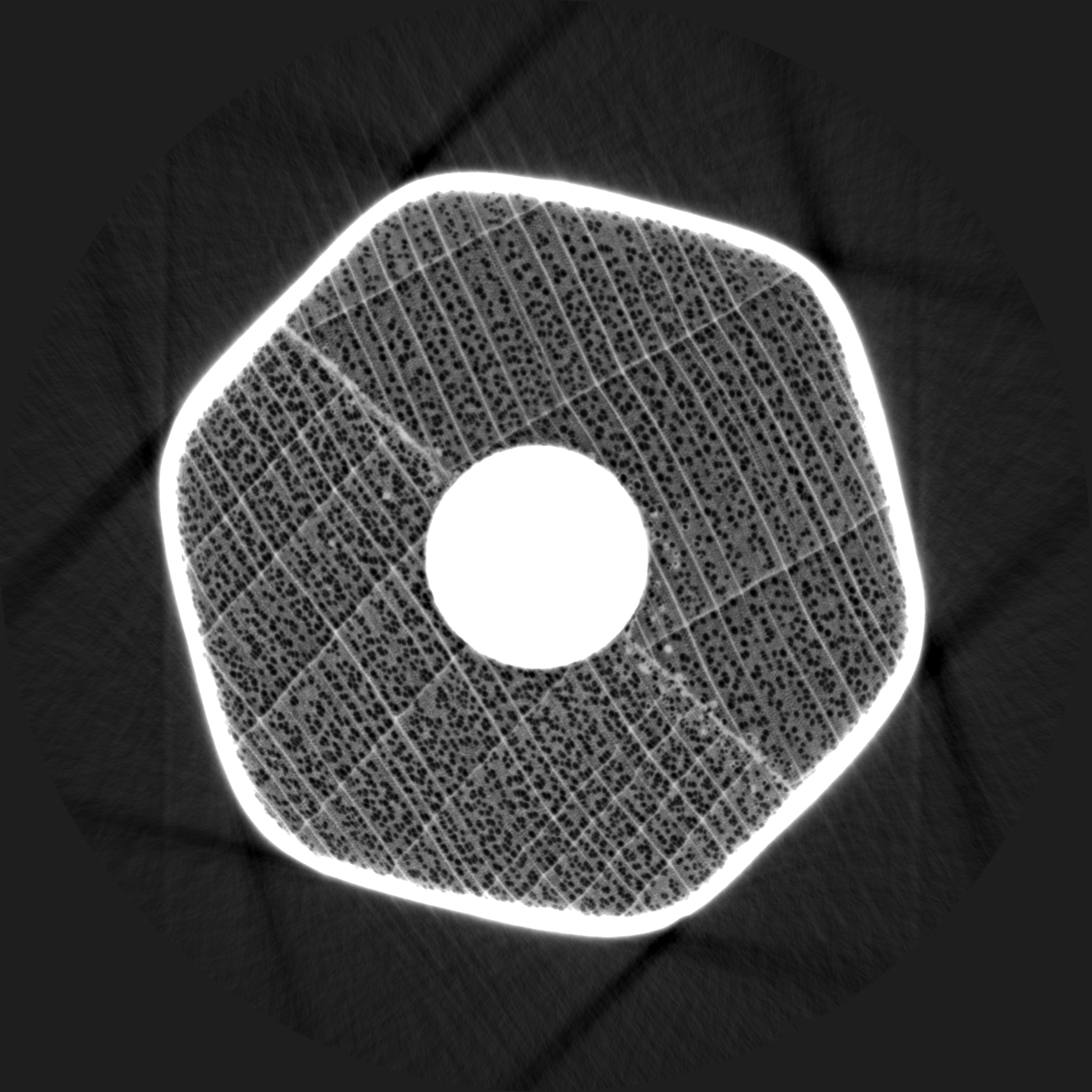# PAGANIN¶

You find the the example in

/scisoft/ESRF_sw/TEST_PYHST/CRAYONInput File:

##
## Input file. It is interpreted as python after a preprocessing
## The preprocessing was made since
##    HST-> PYHST to maintaing compatibility with :
##      a)  NO/YES meaning 0/1  N.A meaning None
##      b)  items containing FILE in their name can be initialized
##          without using the "" which are otherwise necessary for strings
FILE_PREFIX = testsdata/pro_
NUM_FIRST_IMAGE = 0                      # No. of first projection file
NUM_LAST_IMAGE = 1499                    # 1499 No. of last projection file
NUMBER_LENGTH_VARIES = NO
LENGTH_OF_NUMERICAL_PART = 4             # No. of characters
FILE_POSTFIX = .edf
FILE_INTERVAL = 1                        # Interval between input files
NUM_IMAGE_1 = 2048 # Number of pixels horizontally
NUM_IMAGE_2 = 60   # Number of pixels vertically
IMAGE_PIXEL_SIZE_1 = 5.060000 # Pixel size horizontally (microns)
IMAGE_PIXEL_SIZE_2 = 5.060000 # Pixel size vertically
SUBTRACT_BACKGROUND = YES # Subtract background from data
BACKGROUND_FILE = testsdata/dark.edf
# Parameters defining flat-field treatment
CORRECT_FLATFIELD = YES          # Divide by flat-field image
FLATFIELD_CHANGING = YES         # Series of flat-field files
FLATFIELD_FILE = N.A.
FF_PREFIX = testsdata/refHST
FF_NUM_FIRST_IMAGE = 0 # No. of first flat-field file
FF_NUM_LAST_IMAGE = 1500 # No. of last flat-field file
FF_NUMBER_LENGTH_VARIES = NO
FF_LENGTH_OF_NUMERICAL_PART = 4 # No. of characters
FF_POSTFIX = .edf
FF_FILE_INTERVAL = 1500 # Interval between flat-field files
TAKE_LOGARITHM = YES # Take log of projection values
# Parameters defining experiment
ANGLE_BETWEEN_PROJECTIONS = 0.120000 # Increment angle in degrees
ROTATION_VERTICAL = YES
ROTATION_AXIS_POSITION = 1024.285732 # Position in pixels
# Parameters defining reconstruction
OUTPUT_SINOGRAMS = NO # Output sinograms to files or not
START_VOXEL_1 =      1 # X-start of reconstruction volume
START_VOXEL_2 =      1 # Y-start of reconstruction volume
START_VOXEL_3 =   20 # Z-start of reconstruction volume
END_VOXEL_1 =   2048 # X-end of reconstruction volume
END_VOXEL_2 =   2048 # Y-end of reconstruction volume
END_VOXEL_3 =   40 # Z-end of reconstruction volume
OVERSAMPLING_FACTOR = 4 # Oversampling factor : the to-be-backprojected data are oversampled.
# Then nearest neighbour approximation is used. When using GPU
# this parameter has no effect : linear interpolation is done at
# the hardware level
ANGLE_OFFSET = 0.000000 # Reconstruction rotation offset angle in degrees
DO_CCD_FILTER = 1 # CCD filter (spikes)
# Setting this parameter to 1 activates a filtering on each projection. The treatement is applied after :
#      -background subtraction -division by the flat-field -double FF correction ... if these options are activated.
#            CCD_Filter: a median filter is applied on the 3X3 neighbour of every pixel.
#  If the pixel value ( the higher the more photons) exceed the median value more then the threshold
#   parameter then the pixel value is replaced by the median value.
CCD_FILTER = "CCD_Filter"
CCD_FILTER_PARA = {"threshold": 0.040000 }
#     When this option is on (1) a filtering is applied to the sinogramme trying to remove ring artefacts.
#  This options was previously named DO_SINO_FILTER in pyhst1. The old names for this option are still compatible.
#
DO_SINO_FILTER = 0 # Sinogram filter (rings)
SINO_FILTER = "SINO_Filter"
#   old option SINO_FILTER, now can be called "RING_FILTER" : in this case the sinogram is averaged over all the projections, then
#     a high-band pass filter is applied to the average and the result is substracted from all the projections.
#    The details of the frequencies filtering are specified through variable RING_FILTER_PAR
#    More details on the manual : Ring Correction
#
#
#
ar = numpy.ones(2048,'f')
ar=0.0
# ar[2:18]=0.0
xx=numpy.arange(600-2)
ar[2:600]=1.0/(1+numpy.exp((15-xx)/5.0)   )
SINO_FILTER_PARA = {"FILTER": ar }
###
###  DO_AXIS_CORRECTION : When set to 1 ( or YES) this option activates the axis translation correction.
###    The first columns is the horizontal correction. The second , if present, the vertical correction.
###    The datas are the axis displacements. Positive value means positive displacement
###
DO_AXIS_CORRECTION = NO # Axis correction
AXIS_CORRECTION_FILE = correct.txt
#
#
# Parameters for Paganin reconstruction
DO_PAGANIN = 1
PAGANIN_Lmicron = 247.790366
PAGANIN_MARGE = 20
# PAGANIN_MARGE = 600
DO_OUTPUT_PAGANIN = 0
OUTPUT_PAGANIN_FILE = projes/paga_cufft_
PUS=0.0
PUC=0.0
UNSHARP_LoG=0
#
ZEROCLIPVALUE = 0.01 # Minimum value of radiographs after flat / before log
#
# Parameters defining output file / format
OUTPUT_FILE = res2.vol